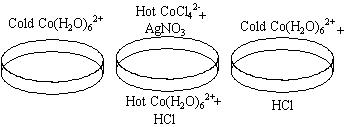## 12.10 The Effect of Temperature on the Co(H2O)62+/CoCl42- Equilibrium

Chemical Concepts Demonstrated: Equilibrium constants relative to temperature, color changes in transition metal complexes, LeChatelier's principle

Demonstration:

 Co(H2O)62+ is formed by dissolving CoCl2*H2O in water. The first sample of the solution is heated to boiling. The first sample now contains a hot CoCl42- solution. AgNO3 is added to produce Co(H2O)62+ again. A second sample is used as a reference. HCl is added to a third sample at room temperature.Observations:

When the solution is heated to boiling, it turns from pink to blue.   This blue solution shifts back to pink as the AgNO3 is added.  When HCl is added to a pink solution, it turns blue.

Explanations (including important chemical equation):

The following equilibrium is observed:

Co(H2O)62+(aq) + 4 Cl-(aq) <=> CoCl42-(aq) + 6 H2O(g)

The Co(H2O)62+ complex is pink, and the CoCl42- complex is blue.

This reaction is endothermic as written, so adding heat causes the equilibrium constant to shift to the right.  This, correspondingly, makes the solution blue.

When the AgNO3 is added, Cl- is removed from solution.  This shifts the equation back to the left, and the solution turns pink again.

When HCl is added, there is more Cl- in solution, so the equilibrium is shifted to the right, and the solution turns blue.

All of the above effects are variations of LeChatelier's principle.